# Chapter 6 Section 5 6 5 Solving Quadratic

• Slides: 16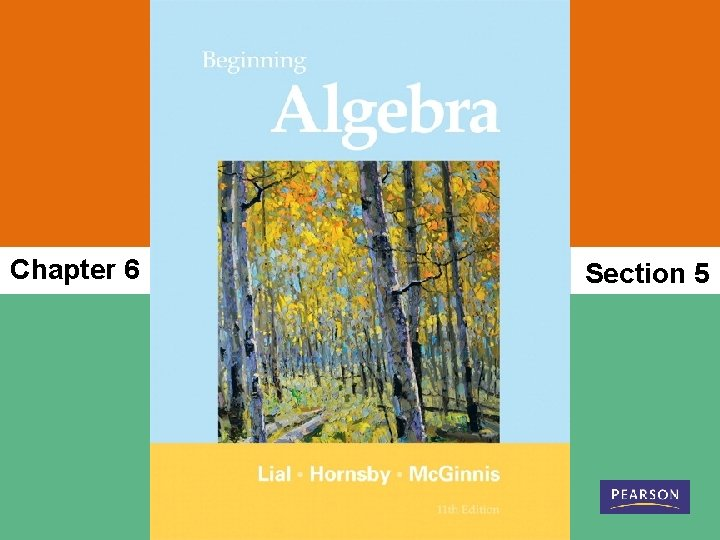Chapter 6 Section 5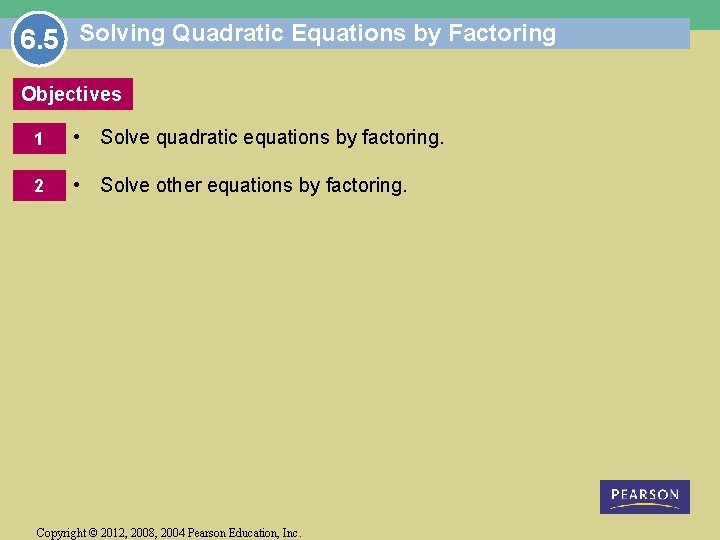6. 5 Solving Quadratic Equations by Factoring Objectives 1 • Solve quadratic equations by factoring. 2 • Solve other equations by factoring. Copyright © 2012, 2008, 2004 Pearson Education, Inc.Solving Quadratic Equations by Factoring. Quadratic Equation A quadratic equation is an equation that can be written in the form ax 2 + bx + c = 0, where a, b, and c are real numbers, with a ≠ 0. The form ax 2 + bx + c = 0 is the standard form of a quadratic equation. For example, and are all quadratic equations, but only x 2 + 5 x +6 = 0 is in standard form. Until now, we have factored expressions, including many quadratic expressions. In this section we see how we can use factored quadratic expressions to solve quadratic equations. Copyright © 2012, 2008, 2004 Pearson Education, Inc. Slide 6. 5 -3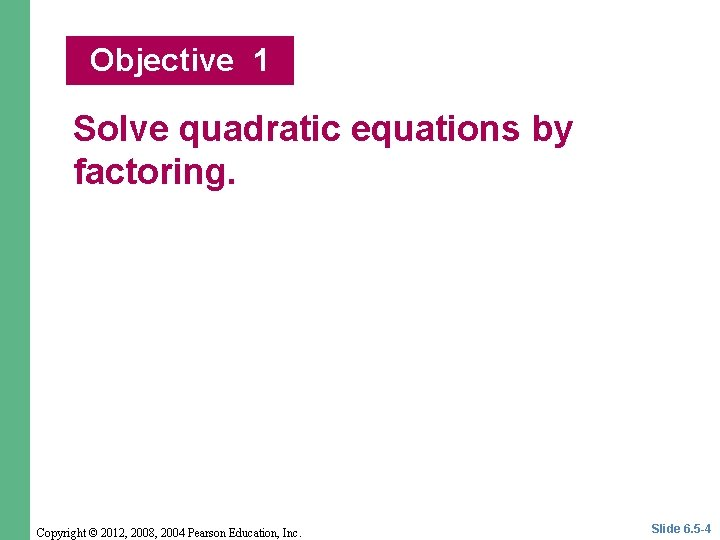Objective 1 Solve quadratic equations by factoring. Copyright © 2012, 2008, 2004 Pearson Education, Inc. Slide 6. 5 -4Solve quadratic equations by factoring. We use the zero-factor property to solve a quadratic equation by factoring. Zero-Factor Property If a and b are real numbers and if ab = 0, then a = 0 or b = 0. That is, if the product of two numbers is 0, then at least one of the numbers must be 0. One number must, but both may be 0. Copyright © 2012, 2008, 2004 Pearson Education, Inc. Slide 6. 5 -5EXAMPLE 1 Using the Zero-Factor Property Solve. Solution: or or Copyright © 2012, 2008, 2004 Pearson Education, Inc. Slide 6. 5 -6EXAMPLE 2 Solving Quadratic Equations Solve. Solution: or or Copyright © 2012, 2008, 2004 Pearson Education, Inc. Slide 6. 5 -7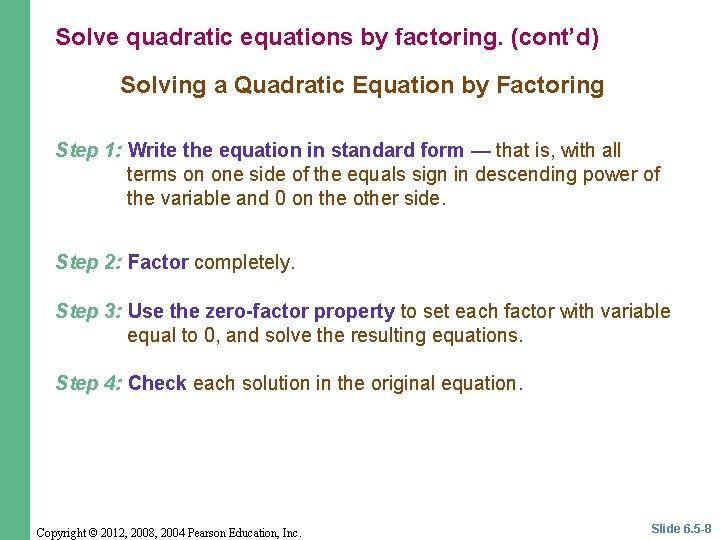Solve quadratic equations by factoring. (cont’d) Solving a Quadratic Equation by Factoring Step 1: Write the equation in standard form — that is, with all terms on one side of the equals sign in descending power of the variable and 0 on the other side. Step 2: Factor completely. Step 3: Use the zero-factor property to set each factor with variable equal to 0, and solve the resulting equations. Step 4: Check each solution in the original equation. Copyright © 2012, 2008, 2004 Pearson Education, Inc. Slide 6. 5 -8EXAMPLE 3 Solving a Quadratic Equation with a Common Factor Solve 3 m 2 − 9 m = 30. Solution: A common error is to include the common factor 3 as a solution. Only factors containing variables lead to solutions. Copyright © 2012, 2008, 2004 Pearson Education, Inc. Slide 6. 5 -9EXAMPLE 4 Solving Quadratic Equations Solve. Solution: Copyright © 2012, 2008, 2004 Pearson Education, Inc. Slide 6. 5 -10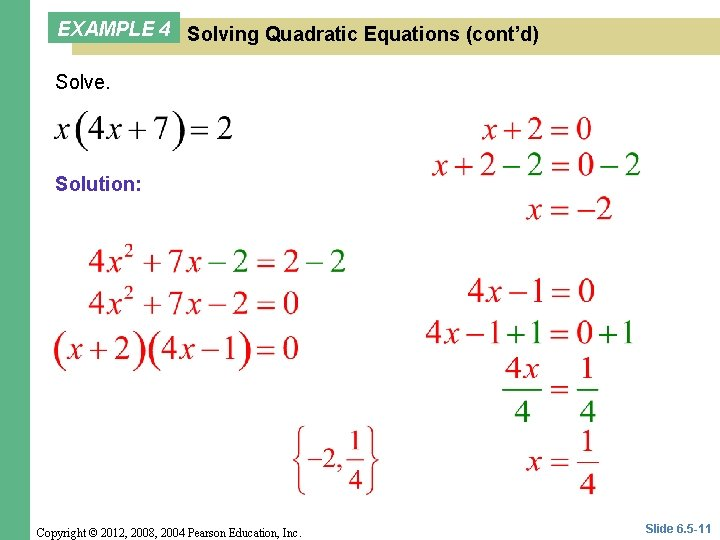EXAMPLE 4 Solving Quadratic Equations (cont’d) Solve. Solution: Copyright © 2012, 2008, 2004 Pearson Education, Inc. Slide 6. 5 -11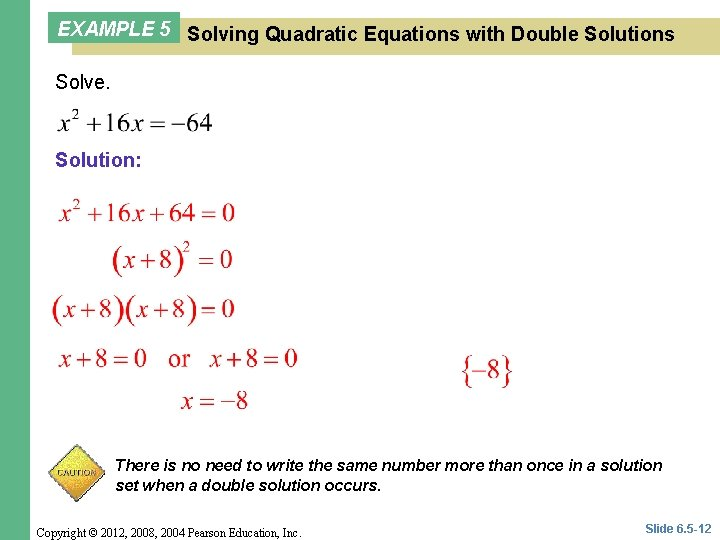EXAMPLE 5 Solving Quadratic Equations with Double Solutions Solve. Solution: There is no need to write the same number more than once in a solution set when a double solution occurs. Copyright © 2012, 2008, 2004 Pearson Education, Inc. Slide 6. 5 -12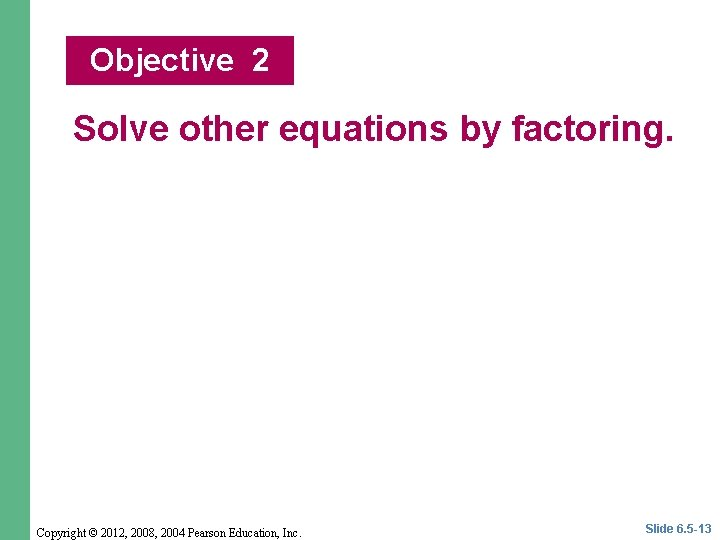Objective 2 Solve other equations by factoring. Copyright © 2012, 2008, 2004 Pearson Education, Inc. Slide 6. 5 -13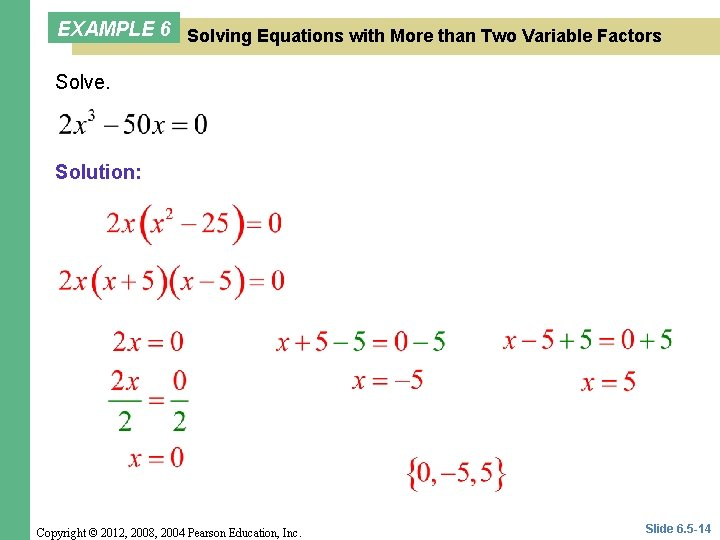EXAMPLE 6 Solving Equations with More than Two Variable Factors Solve. Solution: Copyright © 2012, 2008, 2004 Pearson Education, Inc. Slide 6. 5 -14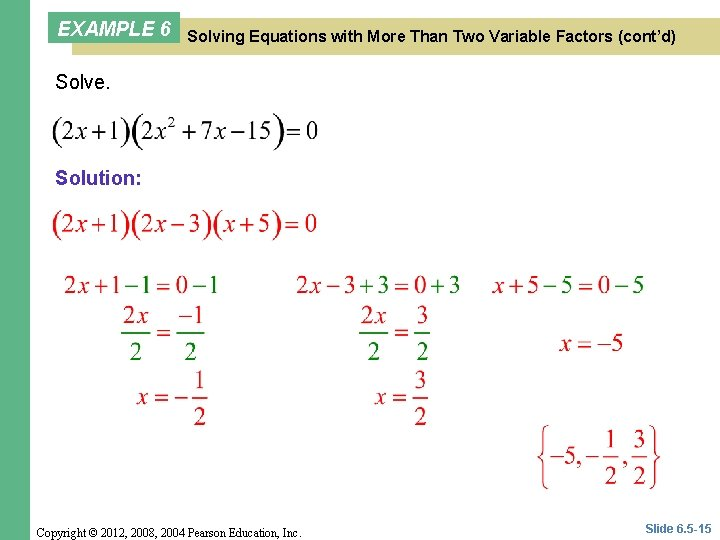EXAMPLE 6 Solving Equations with More Than Two Variable Factors (cont’d) Solve. Solution: Copyright © 2012, 2008, 2004 Pearson Education, Inc. Slide 6. 5 -15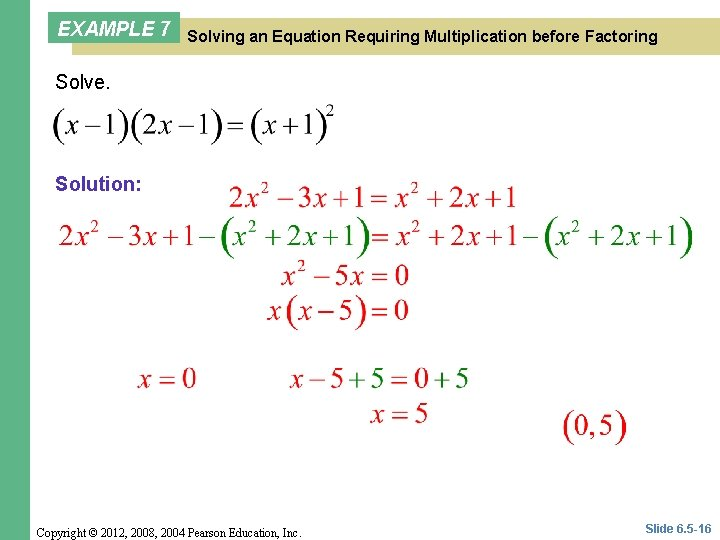EXAMPLE 7 Solving an Equation Requiring Multiplication before Factoring Solve. Solution: Copyright © 2012, 2008, 2004 Pearson Education, Inc. Slide 6. 5 -16